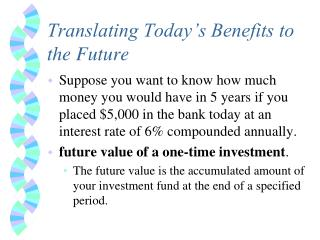# Translating Today’s Benefits to the Future - PowerPoint PPT PresentationDownload PresentationTranslating Today’s Benefits to the Future

Translating Today’s Benefits to the Future
Download Presentation## Translating Today’s Benefits to the Future

- - - - - - - - - - - - - - - - - - - - - - - - - - - E N D - - - - - - - - - - - - - - - - - - - - - - - - - - -
##### Presentation Transcript

1. Translating Today’s Benefits to the Future • Suppose you want to know how much money you would have in 5 years if you placed \$5,000 in the bank today at an interest rate of 6% compounded annually. • future value of a one-time investment. • The future value is the accumulated amount of your investment fund at the end of a specified period.

2. This is an exercise that involves the use of compound interest. • Compound interest - Situation where you earn interest on the original investment and any interest that has been generated by that investment previously. • Earn interest on your interest • First year: \$5,000(1+.06) = \$5,300 • Second year: \$5,300(1+.06) = \$5,618 • Third year: \$5,618(1+.06) = \$5,955.08 • Fourth year: \$5,955.08(1+.06) = \$6,312.38 • Fifth year: \$6,312.38(1+.06) = \$6,691.13

3. Effect of Compound Interest

4. Formula: • FV = PV(1 + r)n • r = interest rate divided by the compounding factor • (yearly r / compounding factor) • n = number of compounding periods • (yearly n * compounding factor) • PV = Present Value of your investment • Compounding Factors: • Yearly = 1 • Quarterly = 4 • Monthly = 12 • Daily = 365

5. Please note that I will always report r’s and n’s as yearly numbers • You will need to determine the compounding factor • All of your terms must agree as to time. • If you are taking an action monthly (like investing every month), then r and n must automatically be converted to monthly compounding. • If you are rounding in time value of money formulas, you need AT LEAST four (4) numbers after the zeros (0) • r = .08/12 • r=0.006667 (not 0.0067 or 0.007 or etc.)

6. Yearly compounding • PV = 5000 • r = .06 • n = 5 • FV = \$5,000(1.06)5 • = \$6,691.13 • Monthly compounding • PV = 5000 • r = (.06/12) = .005 • n = 5(12) = 60 • FV = \$5,000(1+.005)60 • = \$6,744.25

7. How do the calculations change if the investment is repeated periodically? • Suppose you want to know how much money you would have in 24 years if you placed \$500 in the bank each year for twenty-four years at an annual interest rate of 8%. • future value of a periodic investment or future value of an annuity (stream of payments over time) = FVA

8. The formula is... • where PV = the Present Value of the payment in each period • r = interest rate divided by the compounding factor • n = number of compounding periods

9. Let’s try it… • \$500/year, 8% interest, 24 years, yearly compounding • PV = 500 • r = .08 • n = 24 • = 500 (66.7648) • = \$33,382.38

10. Let’s try it again… • \$50/month, 8% interest, 5 years, monthly compounding • PV = 50 • r = (.08/12) = .006667 • n = 5(12) = 60

11. = 50 (73.4769) • = \$3673.84 • Try again with n=120 • FVA=\$9147.30

12. More Practice • You have a really cool grandma who gave you \$1,000 for your high school graduation. You invested it in a 5-year CD, earning 5% interest. How much will you have when you cash it out if it is compounded yearly? • How much will you have if it is compounded monthly? • How much will you have if it is compounded daily?

13. Yearly Compounding • 1000(1+.05)5 • =\$1276.28 • Monthly Compounding • r = (.05/12) = .004167 • n = 5(12) = 60 • 1000(1+.004167)60 • =\$1283.36 • Daily Compounding • r = (.05/365) = .000136986 • n = 5(365) = 1825 • 1000(1+.000136986)1825 • =\$1284.00

14. Some more practice... • You have decided to be proactive for the future, and will save \$25 a month. At the end of 10 years, how much will you have saved, if you earn 8% interest annually? • Monthly Compounding • FVA = • PV = \$25 a month • r = (.08/12) = .006667 • n = (10)(12) = 120 • FVA = \$4573.65

15. Determining when to use Future Value vs. Present Value Calculation/Tables Do I have the money now? No Yes Use PV calculation/table Use FV calculation/table Is it a lump sum? Is it a lump sum? No Yes Yes No Use PV of an annuity Use FV of an annuity Use PV of a single payment Use FV of a single payment

16. Future Value of \$1 (single amount)

17. Future Value of a Series of Annual Deposits (annuity)

18. Present Value of \$1 (single amount)

19. Present Value of a Series of Annual Deposits (annuity)